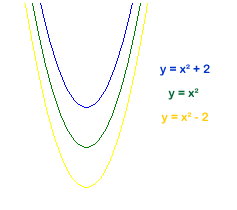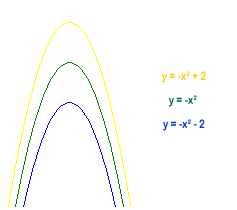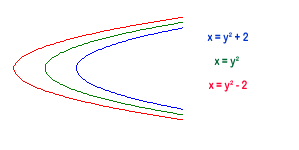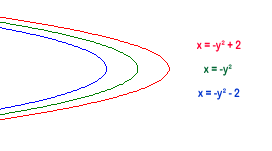#### You may also like### Cubic Spin

Prove that the graph of f(x) = x^3 - 6x^2 +9x +1 has rotational symmetry. Do graphs of all cubics have rotational symmetry?### Sine Problem

In this 'mesh' of sine graphs, one of the graphs is the graph of the sine function. Find the equations of the other graphs to reproduce the pattern.### Parabolic Patterns

The illustration shows the graphs of fifteen functions. Two of them have equations y=x^2 and y=-(x-4)^2. Find the equations of all the other graphs.

# More Parabolic Patterns

##### Age 14 to 18Challenge Level

We have received solutions from Sarah (Madras College, St Andrews), Andrei (School no. 205, Bucharest, Romania) and from Ryan and Belinda (Riccarton High School, Christchurch, New Zealand). Well done to you all.

All approached the problem in a similar way. Ryan and Belinda's response follows:

#### To get the lines curving downward from the top

We are given the equation for the middle curve (that goes through the point $(0,0)$) which is $y=x^2$. From there we add or subtract $2$ to give the curves going through the points $(0,2)$ and $(0,-2)$ respectively, giving the equations -#### To get the lines curving upward from the bottom

This is a reflection of the downward curves, so we use opposite equations, e.g. instead of $y=x^2$we use $y=-x^2$. From there we add or subtract $2$ to give the curves going through the points $(0,2)$ and $(0,-2)$ respectively, giving the equations -#### To get the lines curving from right to left

We are given the equation for the middle curve (that goes through the point $(0,0)$) which is $x=y^2$. From there we add or subtract $2$ to give the curves going through the points $(2,0)$ and $(-2,0)$ respectively, giving the equations -#### To get the lines curving from left to right

This is a reflection of the right to left curves, so we use opposite equations, e.g. instead of $x=y^2$we use $x=-y^2$. From there we add or subtract $2$ to give the curves going through the points $(2,0)$ and $(-2,0)$ respectively, giving the equations -